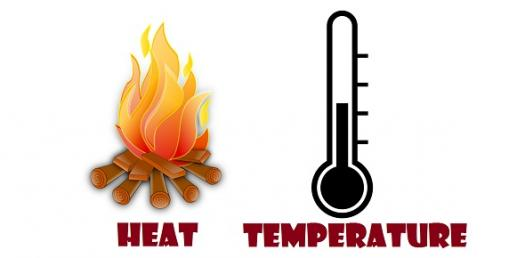# Heat And Temperature Quiz

20 Questions | Total Attempts: 4679Settings.

• 1.
Liquids expand when heated except...
• A.

Alcohol

• B.

Water

• C.

Oil

• D.

Juice

• 2.
Contrast insulators and conductors. Give one example for each.
• 3.
Internal energy is...
• A.

The amount of heat required to change the temperature of something 1 degree C

• B.

The energy transferred between objects because of a temperature difference

• C.

The total of all energies in a substance

• 4.
The ____________ energy is this total amount of energy contained in a(n) _____________.
• 5.
Heat is measured in
• A.

Joules

• B.

Kilograms

• C.

Calories

• D.

A and C

• 6.
Thermal __________ needs to be in contact
• 7.
Calorimeters find the internal energy of something
• A.

True

• B.

False

• 8.
Heat flows hot to cold
• A.

True

• B.

False

• 9.
Heat transfer needs thermal contact
• A.

True

• B.

False

• 10.
What is absolute zero?
• 11.
Specific heat capacity is the amount of heat required to change the temperature of a unit mass of a substance 1 degree C
• A.

True

• B.

False

• 12.
Absolute zeros:
• A.

32 celsius

• B.

72 fahrenheit

• C.

-459 fahrenheit

• D.

-252 celsius

• E.

0 kelvin

• F.

-273 kelvin

• G.

-273 celsius

• H.

100 fahrenheit

• 13.
Heat is the energy transferred between objects because of a ___________________.
• 14.
Things contract when heated because molecules move more
• A.

True

• B.

False

• 15.
Heat is a ______ not a characteristic
• 16.
Describe what happens to an object when heat is added to it.
• 17.
________ is when two objects have the same temperature
• 18.
__________ is when heat flows from one thing to another when touching
• 19.
Temperature is...
• A.

The heat transferred from one thing to another

• B.

How hot or cold something is

• C.

Related to the average molecular kinetic energy in a substance

• D.

Found by two objects in thermal equilibrium

• E.

When particles stop moving

• F.

The measure of kinetic energy of a particle

• G.

Measured in fahrenheit, celsius and kelvin

• H.

Measured in calories and joules

• 20.
Thermal equilibrium has no transfer of energy
• A.

True

• B.

False

Related TopicsBack to top